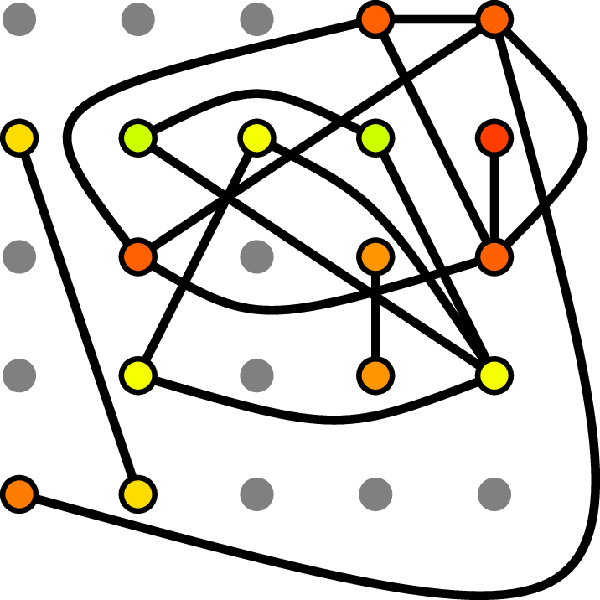# Social percolation revisited: From 2d lattices to adaptive network

## Authors: Frank Schweitzer

Physica A (2021)

### Abstract

The social percolation model (Solomon et al., 2000) considers a 2-dimensional regular lattice. Each site is occupied by an agent with a preference \$x_i\$ sampled from a uniform distribution \$U[0,1]\$. Agents transfer the information about the quality \$q\$ of a movie to their neighbors only if \$x_iłeq q\$. Information percolates through the lattice if \$q=q_c=0.593\$. -- From a network perspective the percolating cluster can be seen as a random-regular network with \$n_c\$ nodes and a mean degree that depends on \$q_c\$. Preserving these quantities of the random-regular network, a true random network can be generated from the \$G(n,p)\$ model after determining the link probability \$p\$. I then demonstrate how this random network can be transformed into a threshold network, where agents create links dependent on their \$x_i\$ values. Assuming a dynamics of the \$x_i\$ and a mechanism of group formation, I further extend the model toward an adaptive social network model.